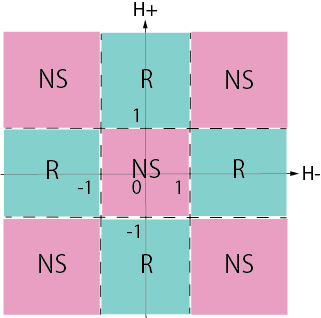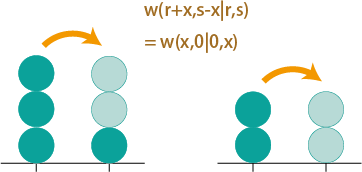Quantum solvable models

My research interest is quantum solvable models and related topics such as spin chains, conformal fields theories, and solvable stochastic processes. Although no clear definition is given for quantum solvable models, here we say a system is solvable when many-body scatterings are decomposed into a sequence of two-body scatterings. The decomposability of many-body scatterings into two-body ones is guaranteed by the Yang-Baxter equation. The Yang-Baxter equation is understood in the context of algebra such as quantum groups, and thus allows us to derive exact physical quantities through the algebraic relations. The famous example is the spin-1/2 Heisenberg chain with anisotropy called the XXZ chain.

Correlation functions of higher-spin XXZ chains

An electron carries either spin 1/2 or -1/2, whereas chemical compounds such as an iron complex may have higher spins, which motivates us to consider spin chains consisting of higher spins. The higher spin chains are also known as integrable systems under special forms of interactions. In the integrable case, the higher-spin model is constructed from the higher-dimensional representation of the Uq(sl2) algebra and therefore the quantum inverse scattering method (QISM) is valid. In the context QISM, physical quantities and eigenstates are expressed by elements of the monodromy matrix. While correlation functions are defined as the expectation values of local operators, the monodromy matrix elements globally act on the spin chain. Correlation functions can be calculated through the commutation relations among the monodromy matrix elements, which are derived from the Yang-Baxter equation.

Correspondence between QFTs and spin chains

A Scattering process of a quantum field theory (QFT) is described by the transfer matrix of the corresponding spin chain. This is achieved by considering the discretization of the light cone, called the light-cone lattice regularization. Due to the construction of the transfer matrix, only limiting excitations of a QFT are obtained from a spin chain under the periodic boundary condition. For instance, in the case of the spin-1 XXZ chain, the corresponding QFT is the supersymmetric (SUSY) sine-Gordon model but only the Neveu-Schwar (NS) sector with the even number of particle can be realized. However, in the presence of boundary magnetic fields corresponding to the Dirichlet boundaries in the QFT, the Ramond (R) sector can be also obtained from the spin chain by manipulating boundary parameters (Fig. 1).Fig. 1 Sector transitions in the UV limit (NS: Neveu-Schwarz, R: Ramond)．

Solvable stochastic processes

The asymmetric simple exclusion process (ASEP) is a one-dimensional stochastic process with discrete space and continuum time. This model obeys a master equation with a Markov matrix, which satisfies the Temperley-Lieb algebra, and thus leads to integrability of the system.

Multi-state ASEPs

Due to the nice mathematical structure of the Markov matrix, various algebraic extensions of the ASEP can be considered. The multi-state extension, which allows more than one particle to occupy the same site, is achieved by considering the higher-dimensional representation of the Temperley-Lieb algebra. Although hopping rules are rather complicated due to the integrability condition, the totally asymmetric limit reduces to a simple model with hopping rates depending only on the number of particles which hop at the same time. (Fig. 2)Fig. 2 Hopping rates of the multi-state TASEP.

2019年度 Aターム

2019年度 Sターム

2018年度 Aターム

2018年度 Sターム